# Numerical Simulation of MILD (Moderate or Intense Low-Oxygen Dilution) Combustion of Coal in a Furnace with Different Coal Gun Positions

Numerical Simulation of MILD (Moderate or Intense Low-Oxygen Dilution) Combustion of Coal in a Furnace with Different Coal Gun Positions

Diego PerroneMario Amelio

Department of Mechanical, Energy and Management Engineering, University of Calabria, Via P. Bucci, Cubo 44/C, 87036 Rende (CS), Italy

Corresponding Author Email:
diego.perrone@unical.it
Page:
S242-S248
|
DOI:
https://doi.org/10.18280/ijht.34S208
|
Accepted:
|
Published:
31 October 2016
| Citation

OPEN ACCESS

Abstract:

The aim of this study is to analyze the temperature field, the specie concentrations of MILD coal combustion in a pilot furnace through the CFD. The attention is focused especially on the effect of the position of coal guns and their distance from the central air jet, in order to investigate both temperature and specie concentration distributions. The authors have performed several simulations in three dimensional steady-state condition of a quarter of the IFRF furnace using a high bituminous A coal. The Eddy Dissipation Model has been used to describe and model the interaction between turbulence and chemistry, while the P1 model has been used for the radiation. In order to describe the solid phase and the combustion process, the Lagrangian description has been used and sub-models have been implemented for devolatilization and char burnout. In particular has been used the Chemical Percolation Model (CPD) for the devolatilization and the intrinsic model for the oxidation of char. The turbulence phenomenon has been modeled resorting to the standard k-ε model, considering the standard wall functions for the wall treatment. The results show that the mild condition is obtained for all three configurations. When the distance between the coal guns and the center air jet are furthest, the oxygen concentration is lowest, consequently the combustion takes place in a fuel-rich zone. This condition can influence the NOx emissions.

Keywords:

MILD, Coal combustion, Computational fluid dynamics.

1. Introduction

The energy consumption is increased because of the rapid industrialization. This energy consumption has caused issues into environment through the emissions of carbon dioxide and nitrogen oxide. In this context, new combustion technologies play an important role in order to contribute to the reduction both pollutant emissions and greenhouse gas (GHG). Under these circumstances, novel approaches of combustion must be studied in order to reduce the pollutant emissions and to increase the efficiency of the fossil fuels combustion.

The dominant energy sources, according to The International Energy Agency’s (IEA’s), remain the fossil fuels like natural gas, coal and oil . Furthermore, the coal, probably, will become the prevalent fuel for the world . The coal is also very abundant, in fact, according to Lackner and Sachs  coal reserves can satisfy the energy needs for the next 100 years.

The Moderate or Intensive Low-oxygen Dilution (MILD) combustion is a new combustion technology, that unlike conventional combustion system, the chemical reactions do not take place in the flame front, but they occur in the whole combustion chamber. This lead to a uniform distribution of temperature and chemical species. Above all peak temperatures are not present, which allow to stabilize the flame, but at the same time, they cause the formation of nitric oxides. The International flame Research Foundation have been carried out experiments on an 880 kW furnace using solid, gaseous and liquid fuels [4-5]. Suda et al.  investigated experimentally the NOx emissions, char burnout, flame stability and ignition delay in a 250 kW furnace. They observed that the peak temperature does not change in a particular range of air-preheated temperature. At the University of Stuttgart have been obtained experimental and numerical results by carrying out measurements and simulations in pilot furnace [7-8]. Schaffel et al.  performed numerical simulations of pulverized coal combustion in pre-heated air condition in a pilot IFRF furnace. Despite they did not use advanced turbulence-chemistry interaction models, their findings, are in agreement with the experimental results. Other authors, such as Vascellari et al. , have analyzed the chemistry-turbulence interaction of MILD pulverized coal combustion modeling by means of CFD. They concluded that advanced and innovative turbulence-chemistry interaction model coupled with detailed chemistry such as, DRM (Direct Reduced kinetic Mechanism), provides better results both for the chemical and fluid dynamic behavior of MILD combustion. Clearly, if an exceptional accuracy is not necessary, a global mechanism is preferred, because it does not need of a high computational cost. The goal of this work is to deepen the behavior of MILD coal combustion, considering different positions of coal gun, in order to explore the temperature and specie concentration fields.

2. Matematical Model

In this work, the CFD ANSYS FLUENT rel. 15.0 has been used to model and predict the thermo-fluid dynamics behavior of MILD combustion. The combustion of coal has been numerically modelled using sub-routines, which describe the phenomena connected to the heating, drying, devolatilization and char burnout. The devolatilization process is modelled by the CPD model, while the Intrinsic model is used for the char combustion.

The coal used in this work is the Venezuelan Guasare coal. The ASTM classifies the Guasare coal as a bituminous coal with a high volatile content. For the numerical description and modeling of the devolatilization process, there are several empirical sub models such as the Kobayashi model . It is an empirical model, which requires empirical input of some parameters related to the kinetic rates and two competing reactions. The CPD model is not an empirical devolatilization model, because the devolatilization behavior is characterized by a rapidly heated coal based on the transformations of the coal structure [12-13-14]. The Figure 1 shows the chemical reaction scheme, which is analogue to the modelling of the cleavage of the bridges and the generation of tar precursors, char and light gas.

## figure_1.png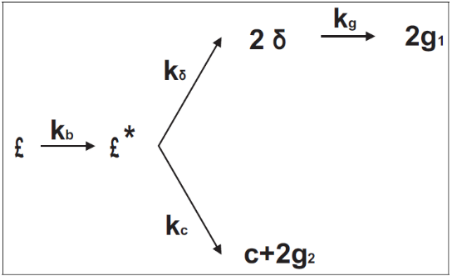Figure 1. Network scheme of chemical bridges for the CPD model 

For an optimal explanation of the CPD theory the authors suggest to consult the Theory’s Guide of Fluent .

Through the C NMR spectroscopy is possible to obtain the chemical structure of the coal . The CPD model requires five input parameters, which can be determined from the C NMR spectroscopy, which is a very complex procedure. Schaffel et al.  utilized the IFRF experimental data in order to characterize both devolatilization and char-combustion processes, and thus determine the five parameters of the CPD model. Since this procedure are expensive and time consuming, in this work has been used the Genetti correlations , which correspond to a series of non-linear correlations able to estimate the five parameters. These parameters are: Mdel, Mcl, σ+1, p0 and c0. The meaning of parameters can be consulted in the Nomenclature. Genetti suggests a quadratic correlation to obtain values for Mdel, Mcl, and σ+1.

$y=c_{1}+c_{2} X_{c}+c_{3} X_{C}^{2}+c_{4} X_{H}+c_{5} X_{H}^{2}+$$c_{6} X_{o}+c_{7} X_{o}^{2}+c_{8} X_{V M}+c_{9} X_{V M}^{2}$(1)

where y = Mdel, Mcl, and σ+1. XC is the mass fractions of Carbon, XH is the mass fraction of Hydrogen, XO is the Oxygen mass fraction, and XVM is the mass fraction of Volatile Matter. In the Schaffel work , the proximate and the ultimate analysis of coal analyzed, are given.

The values of parameters of CPD model, obtained by Genetti correlation and by Schaffel work, are listed in Table 1.

Table 1. CPD model Parameters

 Parameter Symbol Value Unit Cluster molecular weight Side chain molecular weight Lattice coordination number Initial fraction of bridges in coal lattice Initial fraction of char bridges Mcl Mdel σ+1 p0 c0 33.6 359 4.95 0.51 0 Kg/kmol Kg/kmol - - -

The volatiles combustion can be described by two step-chemical mechanisms. It involves the oxidation of the pseudo specie volatile to CO in the first reaction, while in the second reaction the CO is oxidized to CO2:

$C_{133} H_{413} O_{0.5} N_{0.1191}+1.44 O_{2} \rightarrow 1.33 C O+2.06 H_{2} O+0.0595 N_{2}$

$C O+0.5 O_{2} \rightarrow C O_{2}$ (2)

Because the char particles have internal pores, the char oxidation can occur into these pores. Smith and co-workers  have utilized theoretical equations to calculate the rate of char oxidation in the heterogeneous reaction (3). These expressions relate the intrinsic kinetics and gas that surrounds the environment to char reactivity . The intrinsic model is based on the macroscopic pore model, and it is used in this work .

$C(s)+O_{2}(g) \rightarrow C O_{2}(g)$(3)

The input parameters, for this model, are given by the Schaffel work .

In this work the Eddy Dissipation Model , has been chosen to model the chemistry-turbulence interaction. According to the EDM, turbulent mixing controls the rate of the reaction. For this reason is assumed that the Damkholer (Da) number is infinite. The Da number is the ratio between the characteristic mixing scale and the characteristic chemical time. Thus, the kinetic rates can be ignored, and the combustion is based on the mixing.

It is important to note that, for of an assumed reaction, the main idea of the EDM is to consider the turbulent time scale k/ε, instead the chemical time scale. Thereby the model does not consider the effect of chemical kinetics. This model would seem appropriate for this kind of phenomenon as showed by several works [9 - 10].

The turbulence model chosen in this work is the k-ε model .

It is also important to underline that this model do not have an expensive computational cost, but it is very powerful when the chemistry is much faster than the turbulence.

Both the heat of chemical reactions term and the radiation one play an important role in the combustion processes, because they are most responsible of the source term in the energy balance equation. In the furnace of a boiler, the thermal radiation is the prevalent phenomenon of heat transfer. In fact, the flue gas composition influences the radiative heat transfer through the absorption coefficient and emissivity. By solving radiative heat transfer equation (RTE) and by estimating both the radiative gases properties and particles ones it is possible to estimate the radiative heat transfer.

For the absorption coefficient can be used the WSGGM (Weighted-Sum-of-Gray-Gases model) in order to express the absorption coefficient in variable manner. In this work has been used a constant value of 1.5 m-1, along the whole furnace volume. The value has been provided by Schaffel work . The radiation model chosen is the P-1.

The approach used to compute the trajectory of the particles is the Euler-Lagrange approach. The fluid is evaluated as a continuum and it is necessary to solve the Navier-Stokes equations in order to analyze the behavior of fluid. While, by solving the force balance on the coal particles it is possible to predict their trajectories. The particles of coal interact with the gas phase by exchanging momentum, mass and energy. The only forces included in the forces balance are the gravity and the drag forces.

3. Numerical Modeling

The numerical finite volume technique is used to ensure the resolution the governing equations. The CFD code ANSYS® FLUENT, rel. 15.0 is used in order to perform the numerical simulations.

The solver used is the Pressure based for incompressible flow and the algorithm is the segregated solver for the pressure correction and momentum or well known as SIMPLE. For the spatial discretization the interpolation scheme used for the convective term is the upwind scheme, while for the pressure values is the PRESTO! (PREssure Staggering Option) one. To compute the diffusion and convection terms has been used the Least-Squares Cell-Based method.

The axial velocity of the combustive agent injected in the furnace is 65 m/s and the temperature is 1350 °C. The coal velocity, instead, is 22.5 m/s and it is injected at ambient temperature by means of the transport air. The mass flow is 675 kg/h for the comburent and 66 kg/h for the fuel. Consequently, the burner works at 20% excess air in order to give a stoichiometric ratio of λ=1.2. It is necessary point out that the mild combustion condition requires high values of oxidant temperature in order to obtain high temperatures in the combustion chamber. Consequently, in the whole volume, the temperatures of the reactions are above the self-ignition temperature. In a traditional combustion, the reactions are concentrated in the front of the flame.

3.1 Geometry description

The sizes of furnace are the flowing: a square cross-section of 2X2 m and an internal length of 6.25 m. In the Figure 2 is shown the geometry and the position coal injectors. Because of the fluid dynamic and geometry symmetry, a quarter of furnace has been modelled.

The computational domain has been discretized by 590,000 structured cells as shown in the Figure 3. The grid is finer in the region near the coal and air jet.

The number of cells is enough to consider the problem mesh-independence .

## figure_2.png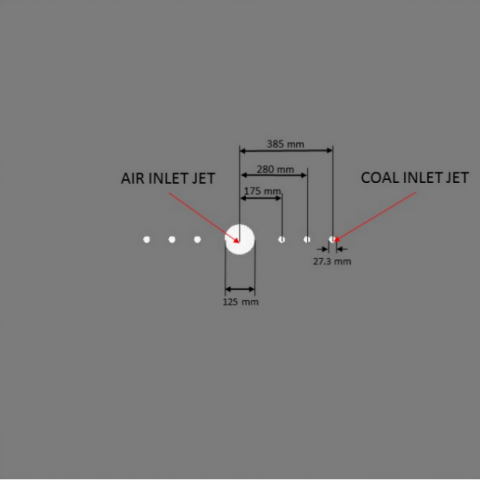Figure 2. Three coal gun configurations

In the prevoius figure is shown the coal gun positions. The configuration of the two coal injectors characterized by three different distances from the oxidant injector. The configuration 1 corresponds to the lowest distance between the coal and air jets, while the configuration 2 and 3 correspond to the medium and greatest distance between the jets. During the experiments were tested two different configurations: low and high velocity of coal. In this work the low-velocity coal gun has been considered, which corresponds to a value of coal diameter to 27.3 mm.

## figure_3.pngFigure 3. Geometry and computational grid of a quarter furnace

4. Results and Discussion

The authors find necessary to show the comparison between the different coal gun positions in order to analyze the temperature field and the specie concentrations. Thus, the aim is understand which configuration is better. In mild combustion several geometrical parameters are very important in order to obtain the “mildness” condition. An important factor is the internal recirculation, which depends on, not only by the high velocity of the jet, but also by the position of it. Consequently, the distance between the coal gun and air jet plays a crucial role. In the following figures will be shown the temperature, the oxygen and carbon dioxide concentration fields. In the Figure 4-5 and 6 are shown the temperature field for the configurations 1-2 and 3, respectively.

## figure_4.jpgFigure 4. Temperature field for the configuration 1

## figure_5.pngFigure 5. Temperature field for the configuration 2

## figure_6.jpg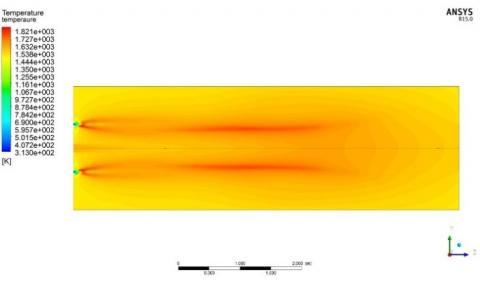Figure 6. Temperature field for the configuration 3

In the configuration 1, the coal and air mix each other rapidly. For this reason, the flame is attached because the combustion takes place in environment with O2 vol % dry higher than 3%. This configuration is closer to a traditional burner than the configuration 3, in which the coal entrains the combustion products. Because the combustion process occurs in a lower O2 % environment the zone becomes fuel-rich. This process, called “intertisation”, allows to obtain values of temperature very similar to the combustion products ones. This is owing to combustion takes place gradually and simultaneously entrains the combustion products at the process temperature. This phenomenon is mainly highlighted in the configuration 3. In the following figures are shown the oxygen concentration fields for the all configurations. It is important to note, downstream of the half furnace, that the concentration of oxygen assumes a uniform distribution. Consequently, the radial profile is flat by assuming a value of about 3 %. The combustion products fills the furnace with the 3 % of O2. In the configuration 3, the ignition stand – off at a distance, form the burner, farther than the configuration 1.

## figure_7.pngFigure 7. Oxygen concentration field for the configuration 1

## figure_8.png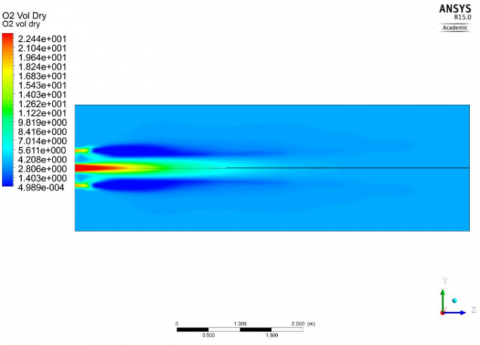Figure 8. Oxygen concentration field for the configuration 2

## figure_9.png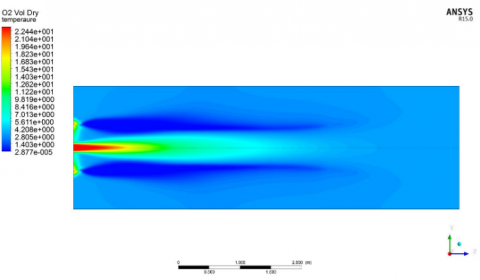Figure 9. Oxygen concentration field for the configuration 3

This result is also highlighted in Figure 10, in which the comparison for the oxygen concentrations along seven radial trasverses is presented.

## figure_10a.jpg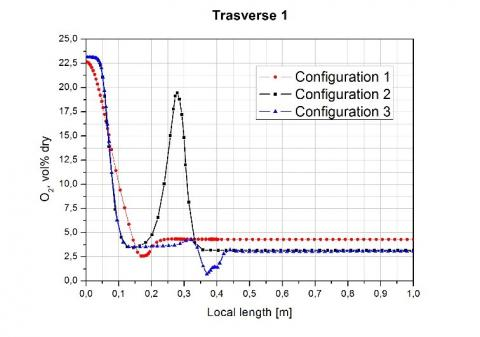## figure_10b.jpg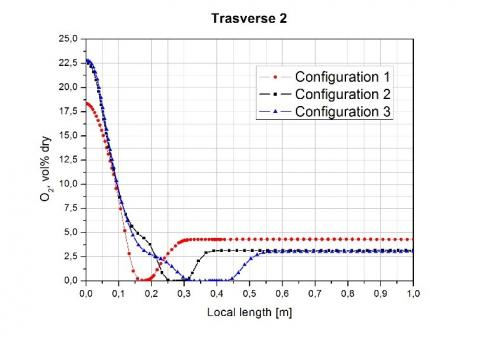## figure_10c.jpg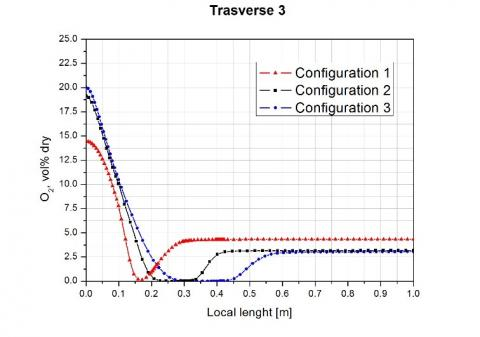## figure_10d.jpg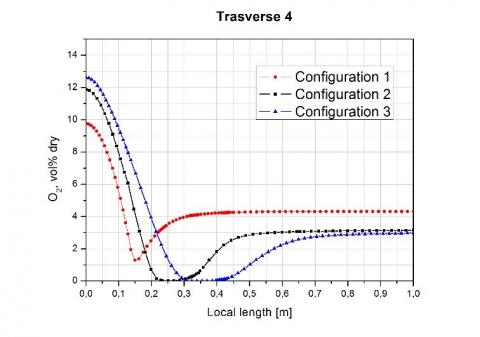## figure_10e.jpg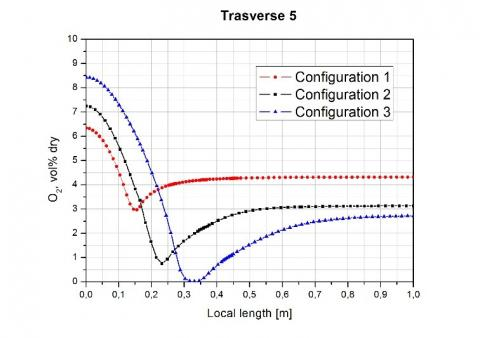## figure_10f.jpg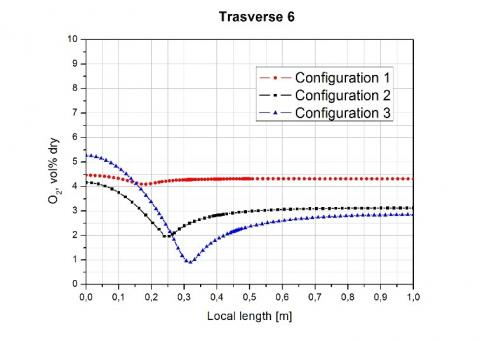## figure_10g.jpg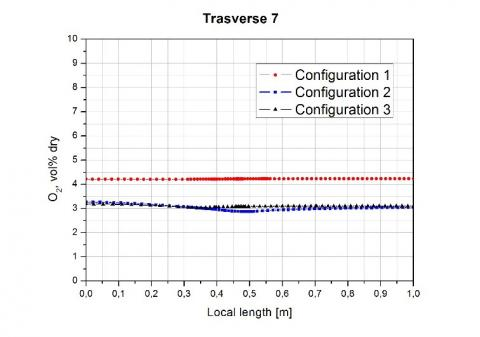Figure 10. Oxygen concentration profiles for different trasverses for all three configurations

The trasverses have been considered at the following distances from the burner: 0.15 m, 0.44 m, 0.735 m, 1.32 m, 2.05 m, 3.22 m and 4.97 m.

It is noteworthy that, in the configuration 1, the oxygen concentration keeps a value higher than 3 % on dry basis.

The carbon dioxide concentration is shown in the following figure.

## figure_11.png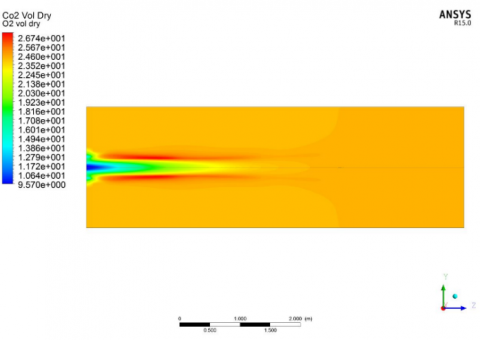Figure 11. Carbon dioxide field for the configuration 1

## figure_12.pngFigure 12. Carbon dioxide field for the configuration 2

## figure_13.png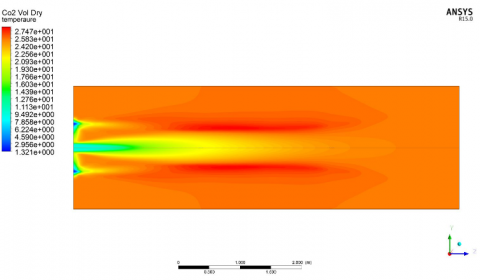Figure 13. Carbon dioxide field for the configuration 3

In Table 4 are summarized the temperature, oxygen and carbon dioxide values at the outlet of furnace for the configurations 1, 2 and 3.

Table 2. Numerical values at the furnace outlet

 Configurations O2 % CO2 % Temperature [K] 1 (175 mm) 2 (280 mm) 3 (385 mm) 4.22 3.31 3.15 24.47 25.38 25.61 1531.5 1534.9 1567.5

The work of Schaffel  provides the experimental results for the configuration 2. The numerical values calculated in this work agree with the measurements.

5. Conclusions

A numerical model has been used to model the combustion phenomenon of coal. Heating, drying, devolatilization and char burnout have been modeled by means of sub-models. The Genetti correlations have been used to calculate the CPD parameters, for the devolatilization sub-routine. The three configurations analyzed, show different temperature and specie concentration fields. The aerodynamic of combustion chamber is very important to get strong recirculation in order to achieve the “mildness” condition. This condition allows to obtain uniform distributions of temperature and combustion products in the whole chamber. It is interesting in study how these distributions affect the NOx emissions, especially the fuel-NOx. The oxygen concentration affects strongly the fuel-NO formation, and they are the 90% of the total NOx formation during the coal combustion . For this reason, the next step of this work is to investigate the NOx emissions in order to understand which configuration provides lower NOx emissions.

Nomenclature
 Mcl Cluster molecular weight, kg. kmol-1 Mdel Side chain molecular weight, kg. kmol-1 σ+1 p0 Dimensionless lattice coordination number Dimensionless initial fraction of bridges in coal lattice c0 Dimensionless initial fraction of char bridges
References

 World Energy Outlook 2010, International Energy Agency, 2010.

 IEA (International Energy Agency), “Energy Technology Perspectives: Scenarios & Strategies to 2050,” Paris, France, 2008.

 K. Lackner and J. Sachs, “A robust strategy for sustainable energy for energy,” Brookings Papers on Economic Activity, vol. 2, pp. 215-284, 2005.

 S. Orsino, M. Tamura, P. Stabat, S. Costantini, O. Prado, R. Weber, Excess enthalpy combustion of coal. Tech. Rep. IFRF Doc. No. f46/y/3, IFRF, 2000.

 Weber R., Smart J., von der Kamp W., “On the (MILD) combustion of gaseous, liquid, and solids fuels in high temperature preheated air,” Proc Combust Inst, vol. 30, no.  2, pp. 2623-9, 2005.

 T. Suda,M. Tajafuji, T. Hirata and J. Stato, “A study of combustion behavior of pulverized coal,” Fuel, vol. 83, pp.  1133-1141, 2004.

 D. Ristic, A. Schuster, G. Scheffknecht, H. Stadler, M. Foerster, R. Kneer and J. G. Wunning, “Experimental study on flameless oxidation of pulverized coal in bench and pilot scale,” Proceeding of the 23th German Flame day, Berlin, Germany, 2007.

 D. Ristic, A. Schuster, G. Scheffknecht, H. Stadler, M. Foerster and J. G. Wunning, “Investigation of NOx formation for flameless coal combustion,” Proceedings of the 7th HiTACG Symposium, Phuket, Thailand, 2008.

 N. Schaffel, M. Mancini, A. Szlek and R. Weber, “Mathematical modeling of MILD combustion of pulverized coal,” Combustion and Flame, vol. 156, pp. 1771-1784, 2009.

 M. Vascellari and G. Cau, “Influence of turbulence-chemical interaction on CFD pulverized coal MILD combustion modeling,” Fuel,  vol. 101, pp. 90-101, 2011.

 H. Kobayashi, J. B. Howard and A. F. Sarofim, “Coal devolatilization at high temperature,” Proc. Combust. Inst., vol. 16, 1976.

 T. H. Fletcher and A. R. Kerstein, “Chemical percolation model for devolatilization: 3. Direct use of 13C NMR data to predict effects of coal type,” Energy and Fuels, vol. 6, pp. 414, 1992.

 T. H. Fletcher, A. R. Kerstein, R. J. Pugmire and D. M. “Grant, chemical percolation model for devolatilization: 2. Temperature and heating rate effects on product yields,” Energy and Fuels, vol. 4, pp. 54, 1990.

 W. L. Flower, R. K. Hanson, C. H. Kruger, 15th Symp. (Int’l) on Combustion, The Combustion Insitute, 823, 1975.

 Fluent Inc. of ANSYS Inc, Fluent 14.5 Documentation. Theory’s Guide, 2012.

 D. Genetti, T. H. Fletcher, R. Pugmire, “Development and application of a correlation of C-13 NMR chemical structural analyses of coal based on elemental composition and volatile matter content,” Energy and Fuels, vol. 13, no. 1, pp. 60-8, 1999.

 I. W. Smith, The combustion rates of coal chars: a review. Symp. Comb. Inst., vol. 19, pp. 1045-1065, 1982.

 B. F. Magnussen and B. H. Hjeritager, “On mathematical model of turbulent combustion with special emphasis on soot formation and combustion,” Symp. Comb. Inst., vol. 16, 1976.

 B. E. Launder and D. B. Spalding, Lectures in Mathematical Models of Turbulence, Academic Press, London, England, 1972.

 C. R. Choeng, N. K. Chang, “Numerical investigation on the flow, combustion and NOx emission characteristics in a 500 MWe tangentially fired pulverized-coal boiler,” Fuel, vol. 88, pp. 1720-1731, 2009.